How Cheenta works to ensure student success?
Explore the Back-Story

# Test of Mathematics Solution Subjective 80 - Inequality of squaresThis is a Test of Mathematics Solution Subjective 80 (from ISI Entrance). The book, Test of Mathematics at 10+2 Level is Published by East West Press. This problem book is indispensable for the preparation of I.S.I. B.Stat and B.Math Entrance.

Also visit: I.S.I. & C.M.I. Entrance Course of Cheenta

## Problem

If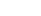are positive numbers, then show that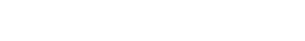.

## Solution

We know that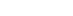L.H.S =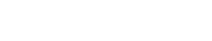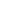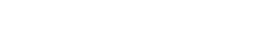[ as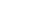,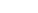,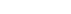&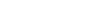for all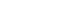]

=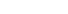= R.H.S [ proved ]This is a Test of Mathematics Solution Subjective 80 (from ISI Entrance). The book, Test of Mathematics at 10+2 Level is Published by East West Press. This problem book is indispensable for the preparation of I.S.I. B.Stat and B.Math Entrance.

Also visit: I.S.I. & C.M.I. Entrance Course of Cheenta

## Problem

Ifare positive numbers, then show that.

## Solution

We know thatL.H.S =[ as,,&for all]

== R.H.S [ proved ]

### Knowledge Partner